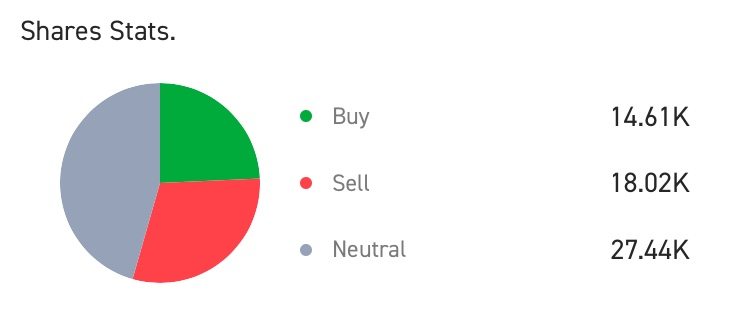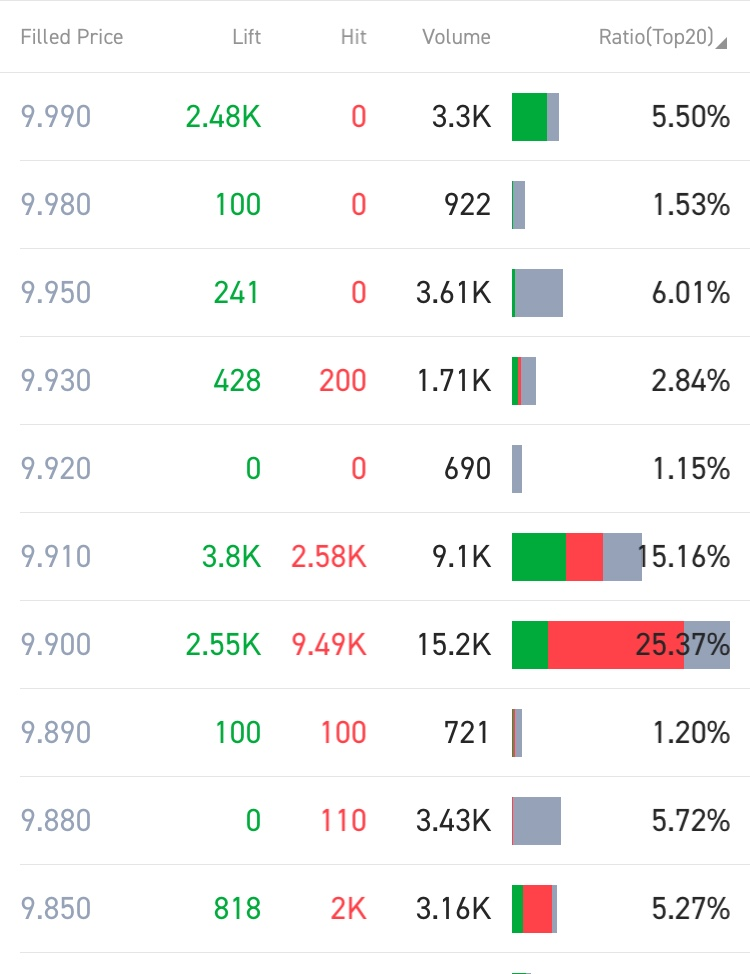Hi,
moomoo ID:0
Log Out
ENGLISH
• ENGLISH
• 中文繁体
• 中文简体

# Introduction to transaction statistics

## 1. Average price

Formula: Average price = total turnover / total volume

Meaning: Refers to the average price of all transactions of a stock that day.

## 2. Total number of transactions

Meaning: Refers to the number of all transactions for a stock that day.

## 3. Total trading volume

Meaning: Refers to the total number of shares (Hong Kong and US stocks)/ lots (A-shares)of a stock traded during the day.

## 4. Statistics of shares/lotsMeaning: By counting the direction of each transaction data and the total volume of the aggregate single direction, users can determine the strength of the day long and short sides.

## 5. Price listMeaning: The price table counts the total number of stocks traded at the same price level, the total number of active buying direction, and the total number of active selling direction according to the price level of each transaction.

## 6. The general analysis points are as follows:

6.1 If the share price is at a high price level, the volume is enlarged, and the share price is on a downward trend that day, it is time to sell.

6.2 If the stock price is at a low price level, the volume is enlarged, and the stock price is on an upward trend that day, it is a buying opportunity.

6.3 If the share price is in a high price band, volume is magnified and the share price is on an upward trend that day, there is still an upward band.

6.4 The stock price is in the low price band and volume is decreasing, indicating an impending reversal.

6.5 The analysis method is not suitable for short term operation.